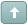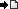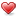Error

 Previous Topic Next Topic
 turtlet #1 Posted : Monday, January 26, 2015 5:07:39 PM(UTC)Rank: NewbieGroups: Registered Users, Subscribers, Unverified UsersJoined: 1/26/2015(UTC)Posts: 6Thanks: 2 times I created a new indicator to give me TRUE when moving average rises continuously for 2 days and false if it does not. I tried to plot it and it works very well. Now when I use the same indicator on the system tester, the buy points generated do not coincide with the indicator. sometimes the system has generated buy points a good 3-4 days after the signal. what am i doing wrong?Wanna join the discussion?! Login to your Discussions forum accountor Register a new forum account.henry1224 #2 Posted : Monday, January 26, 2015 5:18:58 PM(UTC)Rank: Advanced MemberGroups: Registered, Registered Users, SubscribersJoined: 10/29/2004(UTC)Posts: 1,394Location: Glastonbury, CTWas thanked: 1 time(s) in 1 post(s) Without seeing your system code, it is hard to diagnose your problem! As for the system tester, it just determines if your system is profitable!Do not use it for getting tradeable signals! The Expert when attached to a chart will provide you with the actual signals when viewing a chart. the explorer will scan your database for tradeable stocks or signals. The system tester takes whatever data is loaded and uses that particular set to determine profitable systems.If you were to run a system test one day and then run the same test at a different day, you will get 2 different results.turtlet #3 Posted : Monday, January 26, 2015 5:26:17 PM(UTC)Rank: NewbieGroups: Registered Users, Subscribers, Unverified UsersJoined: 1/26/2015(UTC)Posts: 6Thanks: 2 times Originally Posted by: henry1224Without seeing your system code, it is hard to diagnose your problem! As for the system tester, it just determines if your system is profitable!Do not use it for getting tradeable signals! The Expert when attached to a chart will provide you with the actual signals when viewing a chart. the explorer will scan your database for tradeable stocks or signals. The system tester takes whatever data is loaded and uses that particular set to determine profitable systems.If you were to run a system test one day and then run the same test at a different day, you will get 2 different results.   Thanks Henry.  I'm using a system tester to determine the past performance only. Let me elaborate. I created a custom indicator as shown below If(Mov(C,13,E)>Ref(Mov(C,13,E),-1) AND Ref(Mov(C,13,E),-1)>Ref(Mov(C,13,E),-2),1,0) (this gives a TRUE if current peiod moving average is above yesterday's average and if yesterday's average is higher than day befores) Now, when I use the same indicator to check its past performance, the system tester indicates BUY points which do not coincide with the TRUE values from the indicator.mstt #4 Posted : Monday, January 26, 2015 7:25:17 PM(UTC)Rank: Advanced MemberGroups: Registered, Registered Users, SubscribersJoined: 7/25/2005(UTC)Posts: 1,042Was thanked: 57 time(s) in 54 post(s) Hi turtlet If the "system tester" that you're using is the Enhanced System Tester then there is sure to be a good reason why you experience the delayed entry. You haven't provided any setup details for the EST (other than the entry code) so my guess as to the cause of the problem is just that - a guess, but an educated guess all the same. For the most likely scenario I'm going to assume that your entry signal is delayed by one bar. Since your formula relies on the CLOSE it's reasonable to assume that the entry is delayed by 1 bar. I'm also assuming that you're applying 100% of available capital to each trade. The EST calculates the number of shares that can be bought with available capital as at the close of the signal bar, and when the delayed entry price exceeds the price of the bar on which the number of shares was calculated the EST will abort the entry because there isn't enough capital to complete the transaction. Another assumption I'm making is that you're using the "Good until Cancelled" option for your Buy Order, so that when the price drops sufficiently for the order to be filled that's what happens. My solution to this problem is twofold. First, never allocate 100% of capital to any trade. If I wanted a \$1000 trade I'd use settings of \$2000 and apply 50% of capital to the trade. And use a Good for Day order so that all signals are put into effect on the day that they are given. Clearly there are many other issues and peculiarities with the EST but your symptoms appear to fit the the situation I've outlined. However, you should be aware that there are many of other little traps lying in wait for the unwary. Reading the reports the EST produces will sometimes point you directly to the problem. Sadly those same reports often seem meaningless, especially for those unfamiliar with the product. Hope this helps. If you need further help then you should provide ALL the information relating to your system and the EST setup being used. Only then can the problem be properly diagnosed. Royturtlet #5 Posted : Monday, January 26, 2015 8:03:06 PM(UTC)Rank: NewbieGroups: Registered Users, Subscribers, Unverified UsersJoined: 1/26/2015(UTC)Posts: 6Thanks: 2 times Originally Posted by: msttHi turtlet Roy Hi Roy I appreciate you taking your time out to help me out. I had not realised the idiosyncrasies of the system tester so thanks for pointing them out. I made the requisite changes but i still face the same situation. So I'm coming back to you with a more detailed explanation of what im trying to do. I'm not sure if I'm using EST or just a normal ST. My metastock version is 13.0.0702 I built this indicator called (slope of mov avg) and called it in the system tester as shown below...turtlet #6 Posted : Monday, January 26, 2015 8:18:48 PM(UTC)Rank: NewbieGroups: Registered Users, Subscribers, Unverified UsersJoined: 1/26/2015(UTC)Posts: 6Thanks: 2 times i then called this indicator using the  Fml( "slope of Mov Avg") function. I further made the changes by alloting only 50% of available equity as Entry size. and order type as market order--good for day. However i still see a big difference between where a BUY must have been executed and where it is actually treated as being executed. The difference varies between 4-8 days! Im unable to paste my chart here which plots the results on the S&P chart.mstt #7 Posted : Monday, January 26, 2015 10:39:25 PM(UTC)Rank: Advanced MemberGroups: Registered, Registered Users, SubscribersJoined: 7/25/2005(UTC)Posts: 1,042Was thanked: 57 time(s) in 54 post(s) Hi turtlet I can't solve your problem unless ALL system parameters are available. I have to be able to set up the system the way you have before I can track down the problem. The code part is easy; it's one or more of the many other settings that that's the problem. Can you print the System page when looking at results (print to PDF if possible - CutePDF should do that). Printing all other result pages should also work, though from memory I think all formatting of those pages gets lost in when printed. Here's an indicator that plots an equity curve for you system. Getting the EST to plot an identical equity curve is something of a mission but it can be done when both this indicator and the EST are set up appropriately. The Global Variable version of the Trade Equity LE indicator enables the use of the Explorer for multiple securities, and the results can be displayed as an Expert Commentary. email me at rlarsen@quik.co.nz and I'll see what other help I can give. Keep in mind that my time is limited and I'm probably in a quite different time zone as well. The first formula is yours with bits added to provide both entry and exit signals where TE inputs are restricted to just one formula. Roy {Slope of EMA System} {code converted to TE format } M:=Mov(C,13,E)>Ref(Mov(C,13,E),-1) AND Ref(Mov(C,13,E),-1)>Ref(Mov(C,13,E),-2); Entry:=M*Alert(M=0,2); Exit:=(M=0)*Alert(M,2); Entry-Exit; {Trade Equity LE} {Roy Larsen, 2003-2015, 27/1/15, v8.5.4} {Inputs} A:=Input("Trade Equity LE, 0=Long, 100=short, ",0,100,0); B:=Input("Entry, 1=O 2=C 3=H 4=L 5=Stop",1,5,2); Z:=Input("Exit, 1=O 2=C 3=H 4=L 5=Stop",1,5,2); G:=Input("Entry Cost",0,999,0); J:=Input("Exit Cost",0,999,0); D:=Input("Entry/Exit Delays 00-99",0,99,11); K:=1000; F:=-1;{Equity/Factor} {Signals} I:=Fml("Slope of EMA System"); N:=I>0; {Binary entry signal} Ns:= 0; {Price entry signal} X:=I<0; {Binary exit signal} Xs:= 0; {Price exit signal} {Code} Xd:=LastValue(Int(Frac(D/9.9)*10)); D:=LastValue(Int(D/10));Ns:=(B=5)*Ns; F:=ValueWhen(1+D,1,If(Abs(F)>1,Abs(F),-F)); M:=If(Ns>0,Min(H,Max(L,Ns)),If(B=1,O,If(B=3,H,If(B=4,L,C)))); N:=N*(Alert(N=0,2)+(Cum(N>-1)=1)); X:=X*Alert(X=0,2)*(Z<5); N:=ValueWhen(1+D,1,N);N:=If(B<5,N,Ns>0); N:=If((F<=1)*(F>0)*(F*K0)*Min(H,Max(L,Xs)); Y:=If(Xs>0,Xs,If(Z=1,O,If(Z=3,H,If(Z=4,L,C)))); X:=ValueWhen(1+Xd,1,X);X:=X+Xs>0; Y:=If((Z<5)*(X=0),C{Y},Y);Y:=If((Xs>0)*N*X,Xs,Y); I:=Cum(Abs(F)+K+N+X>-1)=1;N:=(I>-1)*N; Y:=If((N+X=0)*Alert(N*X,2),ValueWhen(2,1,Y),Y); R:=BarsSince(I+N)<(BarsSince(I+X)+(Cum(N)=1 AND Cum(I+X)=1)); R:=If((N+X>1)*(Alert(R,2)+((D+Xd<1) *(B<>2)*(Z>1)*(Max(B,Z)>4 OR B<>Z))),1,R); U:=R*Alert(R=0,2)+I;{M:=If(I*(N=0),C,M);} Rx:=Alert(R,2);Q:=Rx*(LastValue(Cum(1))=Cum(1)); Z:={Q+}(R=0)*Rx; D:=A<99; A:=ValueWhen(1,U,If(M,M,1)); F:=ValueWhen(1,U,If(F<0,K*Abs(F), If(F<=1,Int((F*K-G)/A)*A+G,F*A+G)))*(K>0); B:=Rx*(1+BarsSince(U)); I:=(F-G)*Y/A-F;U:=(F-G)*(A-Y)/A-G; N:=(Z+Q>0)*If(D,If(K,I-J*Z,Y-A),If(K,U-J*Z,A-Y)); Xs:=Rx*If(D,If(K,I-Z*J,Y-A),If(K,U-Z*J,A-Y)); X:=Cum((Z+Q>0)*(N>0)*(B-1));Xd:=Cum((Z+Q>0)*(N<=0)*(B-1)); Cum(N)+K+R*(Q=0)*If(D,If(K,I,Y-A),If(K,U,A-Y));1 user thanked mstt for this useful post. turtlet on 1/28/2015(UTC)
 turtlet #8 Posted : Tuesday, January 27, 2015 4:08:53 AM(UTC)Rank: NewbieGroups: Registered Users, Subscribers, Unverified UsersJoined: 1/26/2015(UTC)Posts: 6Thanks: 2 times Originally Posted by: msttHi turtlet I can't solve your problem unless ALL system parameters are available. I have to be able to set up the system the way you have before I can track down the problem. The code part is easy; it's one or more of the many other settings that that's the problem. Can you print the System page when looking at results (print to PDF if possible - CutePDF should do that). Printing all other result pages should also work, though from memory I think all formatting of those pages gets lost in when printed.
 MS Support #9 Posted : Tuesday, January 27, 2015 6:03:55 PM(UTC)Rank: Advanced MemberGroups: Moderators, Registered, Registered Users, SubscribersJoined: 10/8/2010(UTC)Posts: 1,634Thanks: 52 timesWas thanked: 131 time(s) in 127 post(s) Don't forget about "Realistic Market Prices" which delays the execution of all trades to the Open of the bar after the signal. This would be in the "Trade Execution" tab of the System Tester Options (Trade Options button in version 13). One helpful way to investigate these discrepancies can also be to look at the "Orders" tab in your results. You will typically see several states that the trade goes through: Considered Placed Opened Executed / Canceled1 user thanked MS Support for this useful post. turtlet on 1/28/2015(UTC)
 Users browsing this topic
Forum Jump
You cannot post new topics in this forum.
You cannot reply to topics in this forum.
You cannot delete your posts in this forum.
You cannot edit your posts in this forum.
You cannot create polls in this forum.
You cannot vote in polls in this forum.